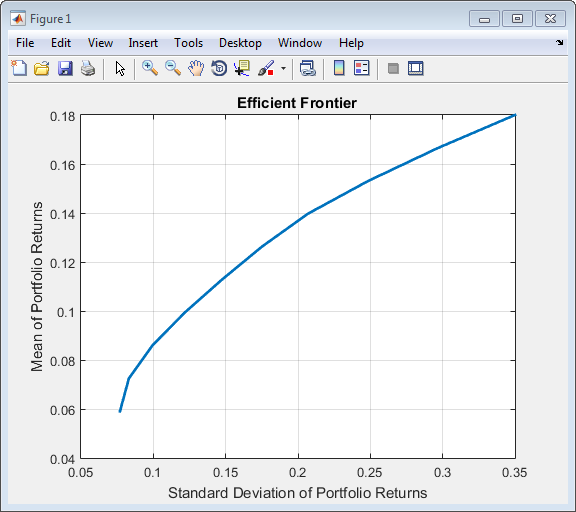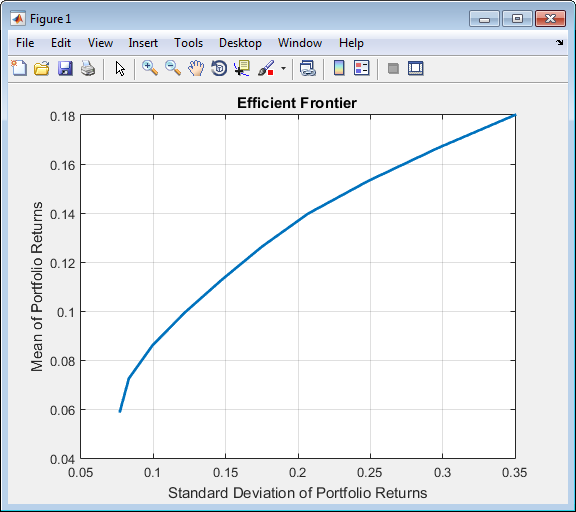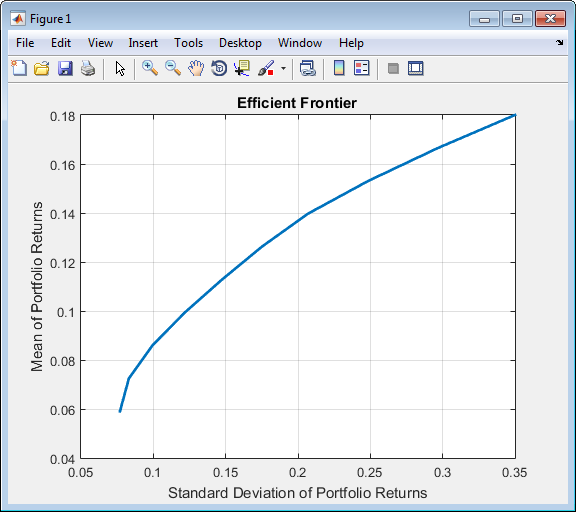## 创建 Portfolio 对象

### 语法

`p = Portfolio;`

`Portfolio` 对象也接受用一系列参数名称-值对组参数指定属性及其值。`Portfolio` 对象接受采用以下一般语法的输入作为公共属性：

` p = Portfolio('property1', value1, 'property2', value2, ... );`

`p = Portfolio(p, 'property1', value1, 'property2', value2, ... );`

`q = Portfolio(p, ...)`

### 投资组合问题充分性

• 必须指定资产收益矩，以使属性 `AssetMean` 包含资产收益的有效有限均值向量，且属性 `AssetCovar` 包含资产收益协方差的有效对称正半定矩阵。

要满足第一个条件，可以设置与资产收益矩相关的属性。

• 可行投资组合集必须是非空紧凑集，其中紧凑集是封闭且有界的。

要满足第二个条件，需要由多个属性定义不同类型的约束以形成一组可行投资组合。由于此类集合必须是有界的，因此可以施加显式或隐式约束，您可以使用 `estimateBounds` 等函数来确保恰当地表示问题。

### Portfolio 函数示例

```p = Portfolio; disp(p) ```
``` Portfolio with properties: BuyCost: [] SellCost: [] RiskFreeRate: [] AssetMean: [] AssetCovar: [] TrackingError: [] TrackingPort: [] Turnover: [] BuyTurnover: [] SellTurnover: [] Name: [] NumAssets: [] AssetList: [] InitPort: [] AInequality: [] bInequality: [] AEquality: [] bEquality: [] LowerBound: [] UpperBound: [] LowerBudget: [] UpperBudget: [] GroupMatrix: [] LowerGroup: [] UpperGroup: [] GroupA: [] GroupB: [] LowerRatio: [] UpperRatio: [] MinNumAssets: [] MaxNumAssets: [] BoundType: [] ```

#### 通过单步设置使用 Portfolio 函数

```m = [ 0.05; 0.1; 0.12; 0.18 ]; C = [ 0.0064 0.00408 0.00192 0; 0.00408 0.0289 0.0204 0.0119; 0.00192 0.0204 0.0576 0.0336; 0 0.0119 0.0336 0.1225 ]; p = Portfolio('assetmean', m, 'assetcovar', C, ... 'lowerbudget', 1, 'upperbudget', 1, 'lowerbound', 0)```
```p = Portfolio with properties: BuyCost: [] SellCost: [] RiskFreeRate: [] AssetMean: [4×1 double] AssetCovar: [4×4 double] TrackingError: [] TrackingPort: [] Turnover: [] BuyTurnover: [] SellTurnover: [] Name: [] NumAssets: 4 AssetList: [] InitPort: [] AInequality: [] bInequality: [] AEquality: [] bEquality: [] LowerBound: [4×1 double] UpperBound: [] LowerBudget: 1 UpperBudget: 1 GroupMatrix: [] LowerGroup: [] UpperGroup: [] GroupA: [] GroupB: [] LowerRatio: [] UpperRatio: [] MinNumAssets: [] MaxNumAssets: [] BoundType: []```
`LowerBound` 属性值进行标量扩展，因为 `AssetMean``AssetCovar` 提供问题的维度。

`p.plotFrontier`#### 通过多步设置使用 Portfolio 函数

```m = [ 0.05; 0.1; 0.12; 0.18 ]; C = [ 0.0064 0.00408 0.00192 0; 0.00408 0.0289 0.0204 0.0119; 0.00192 0.0204 0.0576 0.0336; 0 0.0119 0.0336 0.1225 ]; p = Portfolio; p = Portfolio(p, 'assetmean', m, 'assetcovar', C); p = Portfolio(p, 'lowerbudget', 1, 'upperbudget', 1); p = Portfolio(p, 'lowerbound', 0); plotFrontier(p)``````m = [ 0.05; 0.1; 0.12; 0.18 ]; C = [ 0.0064 0.00408 0.00192 0; 0.00408 0.0289 0.0204 0.0119; 0.00192 0.0204 0.0576 0.0336; 0 0.0119 0.0336 0.1225 ]; p = Portfolio; p = Portfolio(p, 'LowerBound', zeros(size(m))); p = Portfolio(p, 'LowerBudget', 1, 'UpperBudget', 1); p = Portfolio(p, 'AssetMean', m, 'AssetCovar', C); plotFrontier(p)```#### 简写属性名称

`Portfolio` 对象支持部分参数名称简写，以替代 `Portfolio` 对象某些属性的较长参数名称。例如，除了 `'assetcovar'``Portfolio` 对象也支持使用名称 `'covar'`（不区分大小写）设置 `Portfolio` 对象的 `AssetCovar` 属性。每个简写参数名称对应于 `Portfolio` 对象的一个属性。唯一的例外是简写参数名称 `'budget'`，它既可以表示 `LowerBudget` 属性，也可以表示 `UpperBudget` 属性。当使用 `'budget'` 时，则 `LowerBudget``UpperBudget` 属性设置为相同的值以构成等式预算约束。

`ae`

`AEquality`

`ai`

`AInequality`

`covar`

`AssetCovar`

`assetnames``assets`

`AssetList`

`mean`

`AssetMean`

`be`

`bEquality`

`bi`

`bInequality`

`group`

`GroupMatrix`

`lb`

`LowerBound`

`n``num`

`NumAssets`

`rfr`

`RiskFreeRate`

`ub`

`UpperBound`

`budget`

`UpperBudget``LowerBudget`

```m = [ 0.05; 0.1; 0.12; 0.18 ]; C = [ 0.0064 0.00408 0.00192 0; 0.00408 0.0289 0.0204 0.0119; 0.00192 0.0204 0.0576 0.0336; 0 0.0119 0.0336 0.1225 ]; p = Portfolio('mean', m, 'covar', C, 'budget', 1, 'lb', 0); plotFrontier(p) ```

#### 直接设置 Portfolio 对象属性

```m = [ 0.05; 0.1; 0.12; 0.18 ]; C = [ 0.0064 0.00408 0.00192 0; 0.00408 0.0289 0.0204 0.0119; 0.00192 0.0204 0.0576 0.0336; 0 0.0119 0.0336 0.1225 ]; p = Portfolio; p.NumAssets = numel(m); p.AssetMean = m; p.AssetCovar = C; p.LowerBudget = 1; p.UpperBudget = 1; p.LowerBound = zeros(size(m)); plotFrontier(p)```